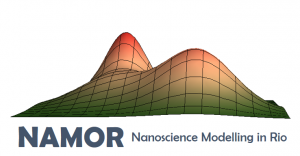# LogoCheck out our new logo and profile picture!

Yes, this is Pão de Açúcar (Sugar Loaf) represented by a mathematical function. Yes, we are nerdy. But we can also be artsy!

In case you were wondering, the Sugar Loaf function is

SL(x,y) = 1 * exp (-((x-0.5)^2 +(y-0)^2)/0.7^2)+1.7*exp (-((x-1)^2 +(y-1)^2)/0.6^2)+2 *exp (-((x+0.3)^2 +(y-0)^2)/0.4^2).

The second term is the Morro da Urca peak and the third is the Pão de Açúcar peak.